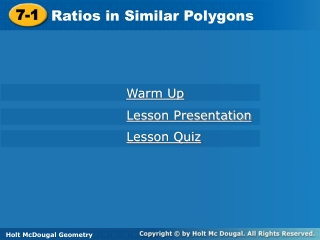DownloadDownload Presentation7-1

# 7-1

Télécharger la présentation## 7-1

- - - - - - - - - - - - - - - - - - - - - - - - - - - E N D - - - - - - - - - - - - - - - - - - - - - - - - - - -
##### Presentation Transcript

1. 7-1 Ratios in Similar Polygons Warm Up Lesson Presentation Lesson Quiz Holt McDougal Geometry Holt Geometry

2. Q  Z; R  Y; S  X; QR  ZY; RS  YX; QS  ZX Warm Up 1.If ∆QRS  ∆ZYX, identify the pairs of congruent angles and the pairs of congruent sides. Solve each proportion. 2.3. x = 9 x = 18

3. Objectives Identify similar polygons. Apply properties of similar polygons to solve problems.

4. Vocabulary similar similar polygons similarity ratio

5. Figures that are similar(~) have the same shape but not necessarily the same size.

6. Two polygons are similar polygons if and only if their corresponding angles are congruent and their corresponding side lengths are proportional.

7. Example 1: Describing Similar Polygons Identify the pairs of congruent angles and corresponding sides. 0.5 N  Q and P  R. By the Third Angles Theorem, M  T.

8. Check It Out! Example 1 Identify the pairs of congruent angles and corresponding sides. B  G and C  H. By the Third Angles Theorem, A  J.

9. A similarity ratiois the ratio of the lengths of the corresponding sides of two similar polygons. The similarity ratio of ∆ABC to ∆DEF is , or . The similarity ratio of ∆DEF to ∆ABC is , or 2.

10. Writing Math Writing a similarity statement is like writing a congruence statement—be sure to list corresponding vertices in the same order.

11. Example 2A: Identifying Similar Polygons Determine whether the polygons are similar. If so, write the similarity ratio and a similarity statement. rectangles ABCD and EFGH

12. Thus the similarity ratio is , and rect. ABCD ~ rect. EFGH. Example 2A Continued Step 1 Identify pairs of congruent angles. A  E, B  F, C  G, and D  H. All s of a rect. are rt. s and are . Step 2 Compare corresponding sides.

13. Example 2B: Identifying Similar Polygons Determine whether the polygons are similar. If so, write the similarity ratio and a similarity statement. ∆ABCD and ∆EFGH

14. Example 2B Continued Step 1 Identify pairs of congruent angles. P  R and S  W isos. ∆ Step 2 Compare corresponding angles. mW = mS = 62° mT = 180° – 2(62°) = 56° Since no pairs of angles are congruent, the triangles are not similar.

15. Check It Out! Example 2 Determine if ∆JLM ~∆NPS. If so, write the similarity ratio and a similarity statement. Step 1 Identify pairs of congruent angles. N  M, L  P, S  J

16. Thus the similarity ratio is , and ∆LMJ ~ ∆PNS. Check It Out! Example 2 Continued Step 2 Compare corresponding sides.

17. Helpful Hint When you work with proportions, be sure the ratios compare corresponding measures.

18. Example 3: Hobby Application Find the length of the model to the nearest tenth of a centimeter. Let x be the length of the model in centimeters. The rectangular model of the racing car is similar to the rectangular racing car, so the corresponding lengths are proportional.

19. Example 3 Continued 5(6.3) = x(1.8) Cross Products Prop. 31.5 = 1.8x Simplify. 17.5 = x Divide both sides by 1.8. The length of the model is 17.5 centimeters.

20. Check It Out! Example 3 A boxcar has the dimensions shown. A model of the boxcar is 1.25 in. wide. Find the length of the model to the nearest inch.

21. Check It Out! Example 3 Continued 1.25(36.25) = x(9) Cross Products Prop. 45.3 = 9x Simplify. 5 x Divide both sides by 9. The length of the model is approximately 5 inches.

22. Lesson Quiz: Part I 1. Determine whether the polygons are similar. If so, write the similarity ratio and a similarity statement. 2. The ratio of a model sailboat’s dimensions to the actual boat’s dimensions is . If the length of the model is 10 inches, what is the length of the actual sailboat in feet? no 25 ft

23. Lesson Quiz: Part II 3. Tell whether the following statement is sometimes, always, or never true. Two equilateral triangles are similar. Always